# Improving the Efficiency of Stand-Alone Solar PV Power Plants

Improving the Efficiency of Stand-Alone Solar PV Power Plants

Artur AkhmetshinMidhat Tuhvatullin Dinar Atnagulov Andrey Linenko Bulat Khalilov

Department of Power Supply and Automation of Technological Processes, Federal State Budgetary Educational Establishment of Higher Education “Bashkir State Agrarian University”, 50-letia Octyabrya Str., 34, Ufa 450001, Russia

Department of Electric Machines and Equipment, Federal State Budgetary Educational Establishment of Higher Education “Bashkir State Agrarian University”, 50-letia Octyabrya Str., 34, Ufa 450001, Russia

Corresponding Author Email:
akhmetshinart6@rambler.ru
Page:
403-412
|
DOI:
https://doi.org/10.18280/ijsdp.160301
30 November 2020
|
Accepted:
4 May 2021
|
Published:
22 June 2021
| Citation

OPEN ACCESS

Abstract:

As a source of alternative energy, solar energy has apparent advantages, including a renewable, inexhaustible, and environmentally friendly resource. However, it has not become widely spread in the Russian Federation. Among the disadvantages of using solar energy are high equipment cost, low efficiency of photovoltaic solar cells, the generated electrical energy instability. The spatio-temporal variability of solar access causes electrical energy instability. It is possible to increase solar photovoltaic plant efficiency by using a tracking system to change the plant sun's spatial orientation. The paper offers mathematical and simulation models of a solar photovoltaic plant with a solar tracking system that allows the plant to be automatically oriented to the sun by matching the production mode and the solar access level. The use of the azimuth plant control system on the sun will increase the power production of the solar PV plant by an average of 28%. The same value will increase by 40% when using the full plant control system.

Keywords:

mathematical model, produced power, solar photovoltaic plant, solar radiation, spatial orientation, tracking system

1. Introduction

One possible way to solve energy and environmental problems related to the energy resources consumption of energy is the use of renewable energy sources, which solar radiation is [1, 2].

Currently, solar energy concentrators and solar tracking systems are the most well-known ways to increase the efficiency of solar PV plants [3, 4].

In order to increase the supply of solar energy to solar photovoltaic cells and reduce its losses on solar PV plants, a constant spatial plant orientation to the solar motion through the sky during light day is necessary. According to the degree of spatial orientation of solar PV plants behind the sun, they are divided into plants with partial and complete orientation. In partial orientation, solar PV plants are installed at a constant angle β to the horizontal, taking into account the sun Azimuth travel (at = a). With this type of orientation, in contrast to the full orientation, there is no complete perpendicularity of the sun rays striking upon the solar photovoltaic plant surface.

Among scientists who studied solar photovoltaic energetics are A.F. Ioffe, D.S. Strebkov, Zh.I. Alferov, V.M. Andreev, V. P. Afanasiev, A. Legue, R.K. Nema, J. Watson, Collados M.V., N.A. Ulapane, etc.

The main ways to increase the efficiency and reduce the cost of solar photovoltaic plants are the use of both solar radiation concentrators and solar tracking systems [5, 6].

There are currently many solar photovoltaic plants that differ in various technical solutions to increase their efficiency factor. The effectiveness of solar photovoltaic plants with solar tracking systems is proved during experiments that are usually carried out using mathematical and simulation modelling [7-9].

Solar photovoltaic plants with a constant orientation are widely used as they are characterized by low cost, simple design, and operation reliability. However, their electrical generation efficiency factor is low due to the non-perpendicular sun rays striking upon the surface of the solar PV array (ξ ≠ 0). Plants with a constant orientation cannot take into account the sun Azimuth travel through the sky. When sun rays are not perpendicular to the solar PV array surface, the incoming solar energy decreases due to its active area reduction, higher losses of solar radiation in protective solar PV array coating, and increased internal energy losses .

The use of concentrators in a solar photovoltaic plant allows:

-increasing the specific solar energy flow falling on solar photovoltaic cells;

-reducing the area of solar photovoltaic cells;

-reducing the solar PV plant cost.

The efficiency of solar radiation conversion of photovoltaic cells can be increased by using concentrated radiation.

The research aims at increasing the efficiency of electric power generated by a stand-alone solar PV plant through its spatial orientation relative to solar radiation, taking into account geographical, climatic, and time factors and the plant technical and design parameters.

Research objectives:

-to develop a mathematical model of a solar PV plant for determining energy characteristics, taking into account its spatial orientation;

-to use the developed mathematical model for creating a simulation solar PV plant model using the MATLAB/Simulink software;

-to study the influence of the solar PV plant spatial orientation on its primary energy characteristics.

2. Methods

Today, most solar PV plants are installed to face a southern direction at a constant angle β to the horizontal (Figure 1).

## 1.jpg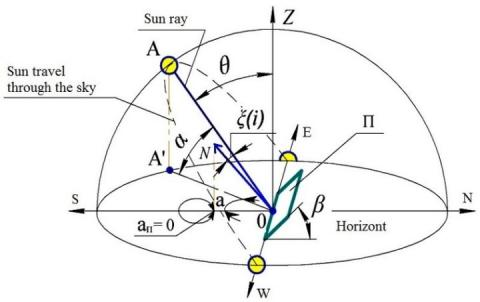Figure 1. Spatial orientation of land-based solar PV plants relative to the sun

Three variants of the solar PV plant spatial orientation are studied in this paper:

-for a solar PV plant with a constant orientation (without a sun tracking system). The solar PV plant is facing a southern direction and installed at an optimal angle to the horizontal β;

-for a solar PV plant with azimuth orientation. The solar PV plant is installed at an optimal angle β to the horizontal and is oriented to the sun by Azimuth;

-a solar PV plant with a full orientation to the sun.

The omnidirectional intensity of solar radiation to the angled surface (in case of constant orientation) is determined by the formula :

$I_{H}=I_{\Pi} \frac{\cos \xi}{\cos \theta}+I_{Д} \frac{(1+\cos \beta)}{2}+\rho\left(I_{\Pi}+I_{Д}\right) \frac{1-\cos \beta}{2}$,   (1)

where, IH- the omnidirectional intensity of solar radiation to the angled surface (in case of constant orientation), W / m2;

$I_{\Pi}, I_{Д}$ are the intensity of the beam and diffuse solar radiation­ to the horizontally located surface, W / m2;

θ- the angle between the directions to the Zenith and the sun, deg.;

$\xi$ - the angle between the normal to the angled surface facing to a southern direction and the direction to the sun, deg.;

β- the angle of the surface slope to the horizontal, deg.;

ρ- the Earth surface reflection coefficient.

Angles θ and $\xi$ are determined by the formulas :

$\cos \theta=\sin \delta \sin \varphi+\cos \delta \cos \varphi \cos \omega$.   (2)

$\cos \xi=\sin (\varphi-\beta) \sin \delta+\cos (\varphi-\beta) \cos \delta \cos \omega$,  (3)

where, δ is the solar declination, deg.;

$\varphi$ - geographical latitude, deg.;

$\omega$ - the solar hour angle, deg.

The solar declination is determined by the following formula [2, 11]:

$\delta=23,45 \cdot \sin \left(360 \cdot \frac{284+n}{365}\right)$,  (4)

where, n is the ordinal number of the day of the year, counted from the first of January.

The formula determines the hour angle:

$\omega=\left(15^{\circ} ч a c^{-1}\right)\left(t_{\text {solar }}-12\right)$,  (5)

where, $t_{\text {solar }}$ is the local time, the hour.

The omnidirectional intensity of solar radiation per plant installed at an angle β and oriented to the sun only by azimuthal bunching ($I_{O R a z}$) was calculated using the formula (1), with the difference in determining the angle between the direction to the sun and the normal.

The formula determines the angle between the direction of the sun and the normal:

$\cos i=\sin \beta\left[\cos \delta\left(\sin \varphi \cos a_{\Pi} \cos \omega+\sin a_{\Pi} \sin \omega\right)-\right.$

$\left.-\sin \delta \cos \varphi \cos a_{\Pi}\right]+\cos \beta[\cos \delta \cos \varphi \cos \omega+\sin \delta \sin \varphi]$.   (6)

where, i the angle between the direction of the sun and the normal, deg.;

$a_{\Pi}$ is the Azimuth of the plant .

For azimuth tracking of the sun, the plant azimuth is equal to the sun azimuth ($a_{\Pi}=a$) (Figure 1), and is determined by the formula:

$a=\arcsin \left(\frac{\cos \delta \sin \varphi}{\cos \alpha}\right)$,  (7)

where, α is the solar altitude angle, deg.,

The formula determines the sun altitude angle (using formula (2)):

$\alpha=\arcsin (\sin \delta \sin \varphi+\cos \delta \cos \varphi \cos \omega) \Rightarrow$.

$\Rightarrow \alpha=\arcsin (\cos \theta)$.   (8)

With the plant full orientation to the sun, the omnidirectional solar radiation intensity is determined by the formula:

$I_{O P}=\frac{I_{\Pi}}{\cos \theta}+I_{\text {Д }} \frac{(1+\cos \theta)}{2}+\rho\left(I_{\Pi}+I_{\text {д }}\right) \frac{1-\cos \theta}{2}$,  (9)

The dependence determined the mathematical regularity of changes in air temperature during the day:

$T_{B}(t)=\bar{T}_{O B}+\frac{\Delta T_{M}}{2} \cos \left(\frac{2 \pi}{t_{\Pi}}\left(t_{\text {solar }}-t_{M}\right)\right)$,  (10)

where, $\overline{T_{O B}}$ is the average daily air temperature, ℃;

$\Delta T_{M}$  - is the daily air temperature amplitude, ℃;

$t_{\Pi}$ - is the period of air temperature change, h;

$t_{M}$ - is the time of occurrence of the maximum value of air temperature at the local solar time, h;

$t_{\text {solar }}$ - local time, h.

The heat generated by a solar PV array depends on the following parameters:

– reflection coefficients of the solar photovoltaic cell;

– efficiency of a solar PV array,

- packing density of solar photovoltaic cells.

The temperature of solar photovoltaic cells is determined using the energy balance equation of a solar PV array using the formula:

$T=\frac{I \cdot\left[\mu-\eta_{0} \cdot\left(1+\chi \cdot T_{0}\right)\right]+\lambda \cdot F \cdot T_{B}}{\lambda \cdot F-I \cdot \eta_{0} \cdot \chi}$,  (11)

where, I is the radiation intensity on solar photovoltaic cells, W / m2;

$\mu$  is the integrated coefficient of solar radiation absorption by a solar PV array;

$\eta_{0}$  is the solar PV array efficiency,

$\chi$  is the temperature gradient depending on the solar PV array type and design;

$T_{0}$  is the solar photovoltaic cell temperature, K;

$\lambda$  is the coefficient of heat transfer from the solar PV array surface, W/(m²· K) ;

F is the ratio of the flat solar PV array area to the illuminated surface area;

TB is the ambient temperature, K.

The formula determines the coefficient of heat transfer from the surface of a solar photovoltaic cell in a linear approximation from the operating temperature of a solar photovoltaic cell:

$\lambda=\lambda_{K}+4 \cdot \varepsilon \cdot \sigma \cdot T_{B}^{3}$,  (12)

where, λK is the convection coefficient, W/(m2·K);

- $\mathcal{E}$  is the integrated coefficient of solar radiation by a solar PV array;

$\sigma$ is the Stefan-Boltzmann constant, W/(m2·K4).

The following parameters are taken into account when calculating the omnidirectional solar radiation intensity:

- angles of sun rays striking upon the receiving surface in direct solar radiation;

- angles of geometric visibility of the receiving surface for diffuse radiation.

There are losses in a solar PV array, which are associated with a protective surface. A solar PV array protective surface is usually made of glass, which has a solar radiation high capacity .

When rays fall perpendicular to the glass surface, the solar radiation transmission coefficient is in the range of 0.9...0.78 The exact value can be obtained by knowing the characteristics of the glass (passport characteristics or reference data) [13, 14].

When the radiation angles are between 0 to 60°, the transmission coefficient has the maximum value. At high angles, the transmission coefficient decreases sharply while the reflection coefficient increases .

Solar energy losses in the protective glass are counted by the solar energy coefficients reflected, absorbed, and transmitted by the glass and their dependence on the sun angle.

The formula determines the transmission coefficient:

$\tau_{n p}=1-\rho_{o m p}$   (13)

where, ρomp is the reflection coefficient.

When the radiation passes through the real environment, part of the radiation is absorbed. Depending on the path travelled in the environment, the radiation attenuation x is described by the Lambert's law :

${{\tau }_{}}=\exp (-x),$   (14)

where, К is the absorption index, K=0.04...0.3 cm-1. The exact value can be obtained by knowing the characteristics of the glass (passport characteristics or reference data)

The path travelled by the sunray in the environment x at a glass thickness B is defined as the trigonometric function argument, cm:

$x=\frac{B}{\cos \Lambda}$,   (15)

where, $\Lambda$ is the angle of the sun rays striking upon the protective glass.

Taking into account the coefficients $\tau_{n p}$ and $\tau_{n p \alpha}$, the actual transmission coefficient is:

$\tau_{\text {nрon }}=\tau_{n p} \cdot \tau_{\text {погл }}$,           (16)

Thus, taking into account the above dependencies, the mathematical model of a solar PV plant has the following form [3, 6]:

\left\{ \begin{align} & {{U}_{}}(T,I,J)=\frac{A\cdot k\cdot T}{q}\cdot \ln \left[ \frac{(I\cdot z-J)({{e}^{\frac{q}{AkT}\left[ {{U}_{{{0}_{XX}}}}-(\frac{I-{{I}_{0}}}{{{I}_{0}}}){{J}_{{{0}_{}}}}\cdot {{R}_{}}+\kappa \cdot \lg (\frac{I}{{{I}_{0}}})+{{U}_{{{0}_{XX}}}}\cdot {{\beta }_{U}}(T-{{T}_{0}}) \right]}}-1)}{I\cdot z}+1 \right]-J{{R}_{}}; \\ & {{J}_{}}(I,T)={{J}_{{{0}_{}}}}+\frac{I-{{I}_{0}}}{{{I}_{0}}}{{J}_{{{0}_{}}}}+{{\beta }_{I}}\cdot {{J}_{{{0}_{}}}}(T-{{T}_{0}}); \\ & T(I,{{T}_{}},\nu )=\frac{{I}'\left[ \mu -{{\eta }_{0}}(1+\chi \cdot {{T}_{0}}) \right]+\left[ (5,7+3,8\nu )+4\varepsilon \cdot \sigma \cdot {{T}_{}}^{3}(n,t) \right]F\cdot {{T}_{}}(n,t)}{\left[ (5,7+3,8\nu )+4\varepsilon \cdot \sigma \cdot {{T}_{}}^{3}(n,t) \right]F-{I}'\cdot {{\eta }_{0}}\cdot \chi }; \\ & I({{I}_{}},{{I}_{}},\rho ,\Lambda \text{,}\Upsilon ,\Omega ,{{\tau }_{}})=\left[ {{I}_{}}(n,t)\frac{\cos \Lambda }{\cos \Omega }+{{I}_{}}(n,t)\frac{(1+\cos \Upsilon )}{2}+\rho (n)\left[ ({{I}_{}}(n,t)+{{I}_{}}(n,t) \right]\frac{(1-\cos \Upsilon )}{2} \right]{{\tau }_{}}; \\ & {{\tau }_{}}(\Lambda )=(1-{{\rho }_{}}(\Lambda ))\exp (-K\frac{B}{\cos \Lambda }); \\ & {{U}_{}}={{N}_{}}\cdot {{U}_{}}(T,I,J); \\ & {{J}_{}}={{N}_{}}\cdot J\cdot {{S}_{}}; \\ & {{P}_{}}={{U}_{}}\cdot {{J}_{}}, \\ \end{align} \right.     (17)

where, $U_{C \Phi э}$ is the solar PV cell voltage, V;

A- non-dimensional curvature parameter of the current-voltage characteristic;

k is the Boltzmann's constant, J/K;

q- electric charge, C (coulomb-volt);

T- the solar PV cell temperature, K;

$I, I^{\prime}$–the intensity of solar radiation striking upon the surface of a solar PV cell and related to the solar PV plant surface unit, W / m²;

z – coefficient of proportionality of the photocurrent density to the solar radiation intensity, A ·m2/W · cm²;

J – current density, A / cm²;

$J_{0_{K 3}}$ – short-circuit current density, A / cm²;

RII–internal electrical resistance, Ohms cm²;

$\boldsymbol{K}$ – the luminosity factor that takes into account the C/V curve shift along the voltage axis, B;

$\beta_{I}, \beta_{U}$– current and voltage temperature coefficients, K-1;

$J_{K 3}$ – short-circuit current density, A / cm²;

$\mu$  is the integrated coefficient of solar radiation absorption by a solar PV array;

$\eta_{0}$  is the efficiency of a solar PV array, %,

$\chi$  is the temperature gradient generally depending on the solar PV array type and design, K-1;

$(5,7+3,8 v)$ – the McAdams dimension ratio for calculating the convection coefficient, W/(m²·K) ,

$v$ - wind speed, m / s;

$\mathcal{E}$  is the integrated coefficient of solar radiation by a solar PV array;

$\sigma$  is the Stefan-Boltzmann constant, W/(m2·K4).

$T_{B}$  is the ambient temperature, K;

n  is the ordinal number of the day of the year;

t  is the time, h;

F  is the ratio of the flat solar PV array surface area (back and face) to the illuminated surface area;

IП, IД is the intensity of the beam and diffuse solar radiation striking upon the horizontally located surface, W / m2 ;

Λ, Ω, Υ – are the angles that determine the spatial orientation of land-based solar PV plants, deg.;

ρ - the Earth surface reflection coefficient;

$\tau_{\text {npon }}, \rho_{\text {omp }}$ – are the coefficients of transmission and reflection of solar radiation by the protective surface of a solar PV array;

$K$ - is the index of solar radiation absorbed by the protective surface of the solar PV array, cm -1;

B is the thickness of the protective surface of the solar PV array, cm;

$U_{C \Phi y}$ – is the solar PV plant voltage, V;

$J_{C \Phi y}$ – is the solar PV plant load current, A;

$N_{\Pi C}, N_{\Pi P}$ – is the number of solar PV cells connected in series and parallel, PCs.;

$S_{C \Phi {\ni}}$ – is the area of the solar PV cell, cm²;

$P_{C \Phi { э }}$ - is the solar PV plant power, W;

The angles that determine the spatial orientation of a land-based solar PV plant for each of the three options are respectively equal:

- for a solar PV plant with a constant orientation (without a sun tracking system). The solar PV plant is facing a southern direction and installed at an optimal angle β to the horizontal;

Λ = ξ, Ω = θ, Υ = β;

- for a solar PV plant with azimuth orientation. The solar PV plant is installed at an optimal angle β to the horizontal and is oriented to the sun by Azimuth;

Λ = i, Ω = θ, Υ = β;

- for a full orientation to the Sun:

Λ = Ω = Υ = θ.

The developed mathematical model makes the following assumptions:

- the series resistance of solar PV cells does not depend on the intensity of solar radiation and temperature;

- the magnitude of the photocurrent is proportional to the intensity of solar radiation and does not depend on temperature;

- the spectral sensitivity of solar PV cells and internal energy losses associated with possible shading of solar PV cells are not taken into account;

- the distribution of solar radiation on the surface of the solar PV array is uniform;

- the temperature of the entire solar PV array volume is assumed to be the same;

- the wind speed for the face and back solar PV array surface is assumed to be the same.

The mathematical model of a solar PV plant allows evaluating the impact of the following factors on the plant output energy characteristics: the degree of the plant orientation on the sun depending on the time of day and year, the intensity of solar radiation, air temperature, and wind speed.

A solar PV plant developed mathematical model is implemented in the MATLAB /Simulink simulation software environment [2, 3].

The simulation model of a solar PV plant consists of three subsystems (Figure 2):

1. “Subsystem 1” reproduces environmental conditions that significantly affect the solar PV plant operation, i.e., air temperature, the intensity of the global solar radiation, taking into account geographical and climatic factors, time of day, day of year, and the degree of spatial orientation of the solar PV plant to the sun.

2. “Subsystem 2” calculates the intensity of solar radiation on the surface of the plant solar photovoltaic cells, considering its design and technological parameters and environmental conditions.

3. “Subsystem 3” calculates and reproduces the output energy characteristics of a solar PV plant. The subsystem makes it possible to calculate and construct the current-voltage and probability-time characteristics of individual solar photovoltaic cells and the solar PV plant as a whole based on internal parameters, such as technological and design plant features and external factors, such as the intensity of solar radiation.

The simulation model of a solar PV plant includes two subprograms:

-a program for calculating the global intensity of solar radiation on a horizontal incline facing a southern direction fully or Azimuth oriented to the solar surface and determining the ambient air temperature;

-computational and graphical program for constructing the energy characteristics of solar photovoltaic cells and a solar PV plant as a whole.

Two connecting blocks are used for the simulation model: a block for calculating a solar photovoltaic cell operating temperature and a block for calculating the loss of solar radiation in a protective glass.

The calculation and graphics program in the MATLAB/Simulink envelop is shown in Figure 3.

## 2.jpg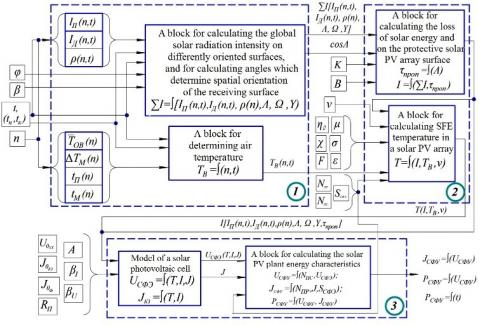Figure 2. Simulation model of a solar PV plant

## 3.jpgFigure 3. Calculation and graphic program for building energy characteristics of solar photovoltaic cells and solar PV plant as a whole

The program is an interrelated algorithm of computational operations. The algorithm calculates and builds the current-voltage and probability-temporal characteristics of both individual solar photovoltaic cells and solar PV plants with different parameters as a whole based on the original data. It also makes it possible to assess the impact on output power characteristics of the internal parameters determined by the properties of the original semiconductor and external factors: solar radiation intensity, number of solar photovoltaic cells in the module connected in series and parallel, and temperature.

The program for calculating the global intensity of solar radiation on a horizontal incline facing a southern direction fully or Azimuth oriented to the sun surface is shown in Figure 4.

The program allows plotting the graph of the global solar radiation intensity on a horizontal incline with a South orientation, fully or azimuthally oriented to the solar surface and ambient air temperature, depending on the day of the year, time of day, and (or) time interval, the geographical latitude of the surface location and its slope angle relative to the horizon.

The initial data set by the program are:

-average daily air temperature,

-daily amplitude, time of maximal temperature occurrence, and period of air temperature change.

-the intensity of the beam and diffuse solar radiation to the horizontally located surface, W / m2;

-the Earth surface reflection coefficient.

## 4.jpg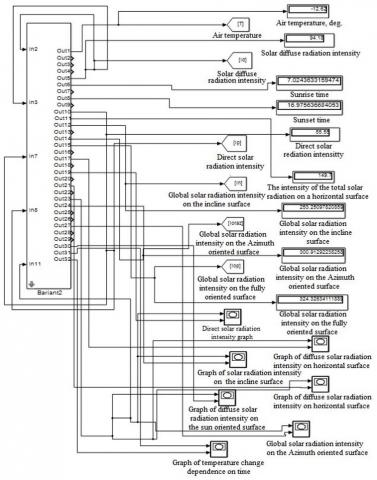Figure 4. Program for calculating the global solar radiation intensity on a horizontal inclined with a South-orientation, fully or azimuthally oriented to the sun surface

3. Research Results

The estimation methods proposed by Fishman and Kiwia were applied to test the developed simulation model [15-17]:

-verification, which can be used to make sure that the model is working as planned;

-assessment of adequacy, i.e., checking the similarity of the model operation with the behaviour of the actual system, which it imitates;

-problem analysis, i.e., establishing statistically significant conclusions based on the results obtained by modelling.

The manufacturer certificate data for specific solar PV plants were compared with the values obtained using “Subsystem 3” and “Computational and graphical program for constructing the energy characteristics of solar photovoltaic cells and solar PV plants as a whole”. The adequacy of the data was evaluated.

The current-voltage and probability-time characteristics were constructed for one solar PV cell and a solar PV array consisting of two modules connected in parallel (each having thirty-six solar photovoltaic cells connected in series) under standard conditions (Figures 5 and 6).

Solar photovoltaic cells made of single-crystalline silicon have the following characteristics:

-short-circuit current density, $J o_{K .3.}$ = 37mA / cm²;

-no-load voltage, $U o_{X X}$ = 0.6 V;

-internal electrical resistance, RP =1Ом·cm²;

-the area of the solar photovoltaic cell, SSFE = 98, 92 cm²;

-current temperature coefficient, $\beta_{I}$ = 0,0006°С-1,

-temperature voltage coefficients, $\beta_{U}$ = – 0,0032°С-1.

-non-dimensional curvature parameter of the current-voltage characteristic, А =1,2.

Solar PV arrays have the following characteristics:

-maximum power, $P_{\max }$  =120W;

- no-load voltage, $U_{X X}$ = 22V;

-short-circuit current density, $J_{K .3 .}$ = 7.34 A;

-optimal operating voltage, $U_{\text {опт }}$ = 18V;

-optimal operating current, $J_{ {OПт }}$ = 6.66 A.

As shown in Figures 5 and 6, the values $U_{X X}$ of $J_{K .3 .}$ both solar PV arrays and solar photovoltaic cells are proportional. The obtained characteristics (Figure 7) coincide with the certificate data of solar PV arrays, confirming the developed mathematical and simulation models adequacy.

The results of the modelling, according to “subsystem 3”, are shown in Figure 7.

Thus, an increase in the solar PV array temperature leads to the no-load voltage and power decrease.

The current-voltage characteristic of a photovoltaic module at different values of the solar radiation intensity on the solar PV array surface is shown in Figure 8.

Reducing solar radiation intensity on the solar PV array surface leads to the current and voltage decrease.

The developed program makes it possible to study the output parameters of a solar PV array in a specific area and select the optimal parameters of a solar PV plant for known values of external factors using a simulation model.

## 5.jpg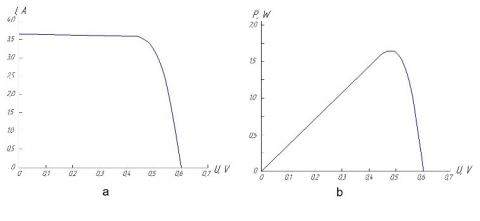Figure 5. Characteristics of solar photovoltaic cells: a-current-voltage characteristic; b – a probability-temporal characteristic

## 6.jpg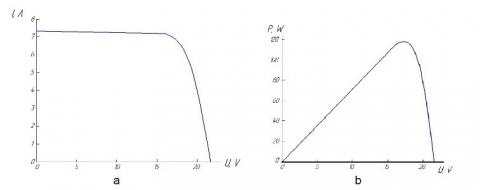Figure 6. Characteristics of solar photovoltaic arrays under standard conditions: a – voltage-current characteristic; b – a probability-temporal characteristic

## 7.jpgFigure 7. Characteristics of solar photovoltaic arrays under standard conditions: temperature: 1 - +40°C; 2 - +10°C; a-current-voltage characteristic; b – probability-temporal characteristic

## 8.jpg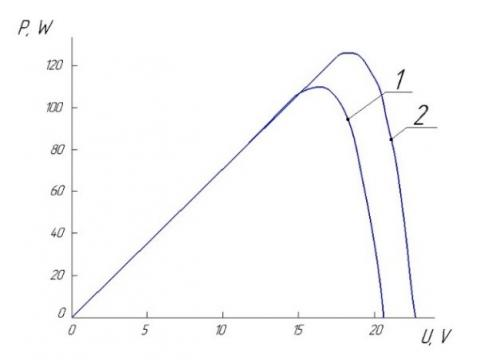Figure 8. Current-voltage characteristic of a photovoltaic module at various values of solar radiation intensity on the solar photovoltaic array surface

4. Practical Application and Results

The main known ways to increase solar PV plant efficiency are developing advanced technologies for manufacturing solar PV plants to reduce their cost, increase efficiency, use solar radiation concentrators, and apply solar tracking systems.

Solar PV plants with constant orientation are widely used due to their simple design, reliability, and relatively low price. However, these plants have a low efficiency of generating electric energy since sun rays fall non-perpendicular to the plant surface (ξ ≠ 0). Moreover, the solar PV plant fixed orientation does not consider the sun Azimuth, and Zenith travel through the sky. Since sun rays fall non-perpendicular to the solar PV plant surface, solar energy entering the plant decreases, which is also due to its active area decrease, an increase in solar radiation losses in the protective coating of a solar PV array, and an increase in internal energy losses caused by individual solar PV plants shading. Papers [11, 15, 18-23] prove this fact.

Using concentrators in solar PV plants increases the solar energy specific flow incident on the solar photovoltaic cell, thereby reducing the solar photovoltaic cell area, and consequently, the cost of solar photovoltaic arrays of the same power. Concentrated radiation increases the efficiency of converting solar radiation from a solar photovoltaic cell, which is also noted in the works of other authors [24-28].

A solar tracking system has been applied to increase the efficiency of stand-alone solar PV plants. The output energy characteristics of a solar PV plant in certain natural and climatic conditions have been determined, considering the influence of the plant spatial orientation relative to the sun and using the developed mathematical and simulation models.

The developed solar PV plant mathematical and simulation models allow determining its energy characteristics based on indicators that consider internal and external factors. Thus, in conditions of the Republic of Bashkortostan, the sun Azimuth orientation in a PV plant increases the solar PV plant capacity by 28% on average. With complete orientation, it is 40% higher compared to the constant orientation.

Acknowledgment

This research did not receive any specific grant from funding agencies in the public, commercial, or not-for-profit sectors. The authors declare that they have no conflict of interests.

Nomenclature
 $I_{\Pi}$ intensity of the beam $I_{Д}$ diffuse solar radiation $\theta$ the angle between the directions to the Zenith and the sun, deg. thermal conductivity, W.m-1. K-1 $\xi$ the angle between the normal to the angled surface facing to a southern direction and the direction to the sun, deg. $\beta$ the angle of the surface slope to the horizontal, deg. $\rho$ the Earth surface reflection coefficient $\delta$ the solar declination, deg. $\varphi$ geographical latitude, deg. $\omega$ the solar hour angle, deg. $t_{\text {solar }}$ the local time, the hour $a_{\Pi}$ the Azimuth of the plant $\alpha$ the solar altitude angle, deg. $\overline{T_{O B}}$ the average daily air temperature, ℃ $\Delta T_{M}$ the daily air temperature amplitude, ℃ $t_{\Pi}$ the period of air temperature change, h $t_{M}$ the time of occurrence of the maximum value of air temperature at the local solar time, h $I$ the radiation intensity on solar photovoltaic cells, W / m2 $\mu$ the integrated coefficient of solar radiation absorption by a solar PV array $\eta_{0}$ the solar PV array efficiency $\chi$ the temperature gradient depending on the solar PV array type and design $T_{0}$ the solar photovoltaic cell temperature, K $\lambda$ the coefficient of heat transfer from the solar PV array surface, W/(m²· * K) $F$ the ratio the flat solar PV array area to the illuminated surface area $T_{B}$ the ambient temperature, K $\lambda_{K}$ the convection coefficient, W/(m2·K) $\rho_{\text {omp }}$ the reflection coefficient
References

 Bezrukikh, P.P., Arbuzov, Yu.D., Borisov, G.A., Vissarionov, V.I., Evdokimov, V.M., Malinin, N.K., Ogorodov, N.V., Puzakov, V.N., Sidorenko, G.I. and Shpak, A.A. (2002). Resources and efficiency of using renewable energy sources in Russia. Nauka, Saint Petersburg, pp. 23-24.

 Iarmukhametov, U.R., Akhmetshin, A.T. (2014). The mathematical model for studying the operation modes of solar plants with photoelectric converters. Energy supply and energy saving in agriculture. Proceedings of the 9th International Scientific and Technical Conference, Moscow, pp. 119-124. https://elibrary.ru/item.asp?id=22029157

 Akhmetshin, A.T. (2016). Improving the efficiency of stand-alone solar PV plants to supply agricultural consumers with electricity. Cand. Tech. Sciences, Saint Petersburg, pp. 1-6. https://elibrary.ru/item.asp?id=27616685

 Lingfors, D., Killinger, S., Engerer, N.A., Widén, J., Bright, J.M. (2018). Identification of PV system shading using a LiDAR-based solar resource assessment model: An evaluation and cross-validation. Solar Energy, 159: 157-172. https://doi.org/10.1016/j.solener.2017.10.061

 Killinger, S., Lingfors, D., Saint-Drenan, Y.M., Moraitis, P., van Sark, W., Taylor, J., Engerer, N.A., Bright, J.M. (2018). On the search for representative characteristics of PV systems: Data collection and analysis of PV system azimuth, tilt, capacity, yield and shading. Solar Energy, 173: 1087-1106. https://doi.org/10.1016/j.solener.2018.08.051

 Yang, D., Bright, J.M. (2020). Worldwide validation of 8 satellite-derived and reanalysis solar radiation products: A preliminary evaluation and overall metrics for hourly data over 27 years. Solar Energy, 210: 3-19. https://doi.org/10.1016/j.solener.2020.04.016

 Akhmetshin, A.T. (2014). Mathematical model of a photovoltaic module in the MATLAB/SIMULINK software environment. In: Current problems of agricultural energy. Materials of the V International Scientific and Practical Conference, pp. 34-40. https://elibrary.ru/item.asp?id=21549844

 Mason, K., Reno, M.J., Blakely, L., Vejdan, S., Grijalva, S. (2020). A deep neural network approach for behind-the-meter residential PV size, tilt and azimuth estimation. Solar Energy, 196: 260-269. https://doi.org/10.1016/j.solener.2019.11.100

 Collados, M.V., Chemisana, D., Atencia, J. (2016). Holographic solar energy systems: the role of optical elements. Renewable and Sustainable Energy Reviews 59: 130-140. https://doi.org/10.1016/j.rser.2015.12.260

 Akhmetshin, A.T., Lukmanov, R.L. (2013). Development of an algorithm for moving the receiving unit in the design of systems with a fixed solar energy concentrator. Fundamentals of scientific and technical and technological modernization of the agro-industrial complex: materials of the All-Russian Scientific and Practical Conference, pp. 370-375. https://elibrary.ru/item.asp?id=20722071

 Tsai, H.L., Tu, C.S., Su, Y.J. (2008). Development of generalized photovoltaic model using MATLAB/ Simulink. In: Proceedings of the World Congress on Engineering and Computer Science, pp. 110-116.

 Iarmukhametov, U.R., Akhmetshin, A.T. (2014). Simulation of operating modes of solar installations with photoelectric converters depending on internal and external factors in the MATLAB/SIMULINK environment. Vestnik Krasnoiarsk State University of Agriculture, 8(95): 195-200. https://elibrary.ru/item.asp?id=21989512

 Feng, C., Yang, D., Hodge, B.M., Zhang, J. (2019). OpenSolar: Promoting the openness and accessibility of diverse public solar datasets. Solar Energy, 188: 1369-1379. https://doi.org/10.1016/j.solener.2019.07.016

 Bruder, F.K., Fäcke, T., Rölle, T. (2017). The chemistry and physics of bayfol® HX film holographic photopolymer. Polymers, 9(10): 472. https://doi.org/10.3390/polym9100472

 AlSkaif, T., Dev, S., Visser, L., Hossari, M., van Sark, W. (2020). A systematic analysis of meteorological variables for PV output power estimation. Renewable Energy, 153: 12-22. https://doi.org/10.1016/j.renene.2020.01.150

 Chantana, J., Mano, H., Horio, Y., Hishikawa, Y., Minemoto, T. (2017). Spectral mismatch correction factor indicated by average photon energy for precise outdoor performance measurements of different type photovoltaic modules. Renewable Energy, 114: 567-573. https://doi.org/10.1016/j.renene.2017.07.061

 Nishioka, K., Miyanuma, K., Ota, Y., Akitomi, M., Chiba, Y., Masuda, A. (2018). Accurate measurement and estimation of solar cell temperature in photovoltaic module operating in real environmental conditions. Japanese Journal of Applied Physics, 57(8S3): 08RG08.

 Pylypova, O.V., Evtukh, A.A., Parfenyuk, P.V., Korobchuk, I.M., Havryliuk, O.O., Semchuk, O.Y. (2018). Influence of Si nanowires on solar cell properties: effect of the temperature. Applied Physics A, 124(11): 1-7. https://doi.org/10.1007/s00339-018-2200-6

 Watson, J., Schmela, M. (2018). Global Market Outlook for Solar Power 2018 - 2022. Solar Power Europe. http://www.solarpowereurope.o rg/wp-content/uploads/2018/09/Global-Market-Outlook-2018-2022.pdf, accessed on Oct. 13, 2020.

 Semenenko, M., Kyriienko, O., Yilmazoglu, O., Steblova, O., Klyui, N. (2014). Photo-assisted field emission and electro-reflectance modulation investigations of GaN nanorod arrays. Thin Solid Films, 564: 218-221. https://doi.org/10.1016/j.tsf.2014.06.017

 Lukmanov, R.L., Galimardanov, I.I., Akhmetshin, A.T. (2013). Development of a method for calculating the energy indicators of a stationary solar energy concentrator. Proceedings of the International Academy of Agricultural Education, 17: 249-252. https://elibrary.ru/item.asp?id=19318743

 Bensalem, S., Chegaar, M., Herguth, A. (2017). Band gap dependence with temperature of semiconductors from solar cells electrical parameters. Current Applied Physics, 17(1): 55-59. https://doi.org/10.1016/j.cap.2016.10.012

 Volkova, E.B., Remennikova, I.A., Vecherinina, E.A. (2019). Structural and semantic features of three-component complex sentences of successive subordination in Russian and Romano-Germanic mathematical texts. International Journal of Engineering and Advanced Technology, 8(4): 1086-1090.

 Nema, R.K., Nema, S., Agnihorti, G. (2009). Computer simulation base study of photovoltaic cell/modules and their experimental verification. International Journal of Recent Trends in Engineering, 1(3): 151-156.

 Ortega, E., Aranguren, G., Jimeno, J.C. (2019). New monitoring method to characterize individual modules in large photovoltaic systems. Solar Energy, 193: 906-914. https://doi.org/10.1016/j.solener.2019.09.099

 Saracoglu, B. (2018). Solar star projects SAM version 2017.9.5 PVwats version 5 model case study & validation. International Journal of Energy Applications and Technologies, 5(1): 13-28. https://doi.org/10.31593/ijeat.403748

 Evtukh, A., Grygoriev, A., Litovchenko, V., Steblova, O., Yilmazoglu, O., Hartnagel, H. L., Mimura, H. (2014) Influence of the emitted electron energy distribution from nanocathodes upon the current–voltage characteristics. Journal of Vacuum Science & Technology B, Nanotechnology and Microelectronics: Materials, Processing, Measurement, and Phenomena, 32(2): 02B104. https://doi.org/10.1116/1.4843715

 Hishikawa, Y., Takenouchi, T., Higa, M., Yamagoe, K., Ohshima, H., Yoshita, M. (2019). Translation of solar cell performance for irradiance and temperature from a single IV curve without advance information of translation parameters. IEEE Journal of Photovoltaics, 9(5): 1195-1201. https://doi.org/10.1109/JPHOTOV.2019.2924388